Math Skills ReviewDimensional Analysis

Dimensional Analysis (also called Factor-Label Method or the Unit Factor Method) is a problem-solving method that uses the fact that any number or expression can be multiplied by one without changing its value. It is a useful technique. The only danger is that you may end up thinking that chemistry is simply a math problem - which it definitely is not.

Unit factors may be made from any two terms that describe the same or equivalent "amounts" of what we are interested in. For example, we know that

1 inch = 2.54 centimeters
Note: Unlike most English-Metric conversions, this one is exact. There are exactly 2.540000000... centimeters in 1 inch.

We can make two unit factors from this information: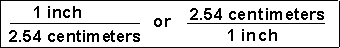Now, we can solve some problems. Set up each problem by writing down what you need to find with a question mark. Then set it equal to the information that you are given. The problem is solved by multiplying the given data and its units by the appropriate unit factors so that only the desired units are present at the end.

(1) How many centimeters are in 6.00 inches?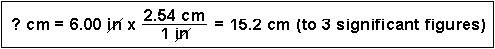(2) Express 24.0 cm in inches.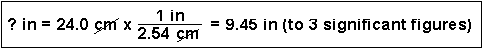You can also string many unit factors together.

(3) How many seconds are in 2.0 years?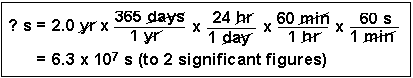Scientists generally work in metric units. Common prefixes used are the following:
PrefixAbbreviationMeaningExample
mega-M1061 megameter (Mm) = 1 x 106 m
kilo-k1031 kilogram (kg) = 1 x 103 g
centi-c10-21 centimeter (cm) = 1 x 10-2 m
milli-m10-31 milligram (mg) = 1 x 10-3 g
micro-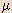10-61 micrometer (g) = 1 x 10-6g
nano-n10-91 nanogram (ng) = 1 x 10-9 g

(4) Convert 50.0 mL to liters. (This is a very common conversion.)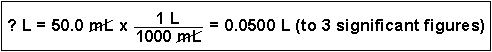(5) What is the density of mercury (13.6 g/cm3) in units of kg/m3?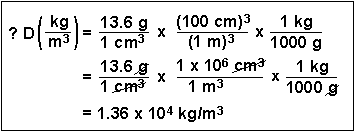We also can use dimensional analysis for solving problems.

(6) How many atoms of hydrogen can be found in 45 g of ammonia, NH3?
We will need three unit factors to do this calculation, derived from the following information:

1. 1 mole of NH3 has a mass of 17 grams.
2. 1 mole of NH3 contains 6.02 x 1023 molecules of NH3.
3. 1 molecule of NH3 has 3 atoms of hydrogen in it.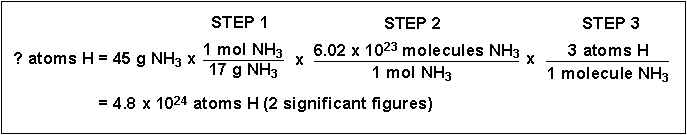There are many, many more examples in your textbook!

QUIZ:
 Question 1 How many millimeters are present in 20.0 inches? Question 2 The volume of a wooden block is 6.30 in3. This is equivalent to how many cubic centimeters? Question 3 A sample of calcium nitrate, Ca(NO3)2, with a formula weight of 164 g/mol, has 5.00 x 1027 atoms of oxygen. How many kilograms of Ca(NO3)2 are present?

Answers: (1) 508 mm (2) 103 cm3 (3) 227 kgAlgebraic ManipulationSignificant FiguresManipulation of ExponentsScientific NotationLogarithmsThe Quadratic Equation